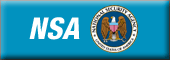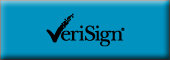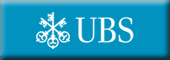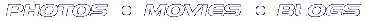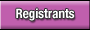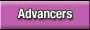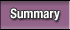See more photos!Petr is the new Algorithm ChampionDiscuss this match
Friday, May 5, 2006
Introduction by lbackstrom

Here at the Aladdin, the excitement has been building all week up to today's big event. While Petr vs Tomek may not be quite as big as Holyfield vs. Tyson, it's certainly right up there.

The competitors started their prep-phase promptly at 2 PM. Some of the competitors spent the whole half hour pecking furiously away at their template code, while others, like Petr, were confident that they could win without fancy macros and prewritten functions.

The round started out fast, as the competitors breezed through the easy problem, arguably the easiest so far. The medium problem slowed them down a bit, but many of the competitors managed to solve it for solid scores. John Dethridge characteristically skipped on to the hard, but eventually went back to the medium.

The straightforward easy problem even left many of the competitors enough time to take a stab at the hard, and by the end of the coding phase, 4 competitors had submitted all 3 problems. The tight race (and crowd knowledge of many bad solutions) ensured that the challenge phase would be particularly exciting, and it did not disappoint.

Petr struck first, shooting down three medium problems in the first few seconds. However, his next two challenges failed, and he ended up with a net of 100 points. fuwenjie, the underdog of the match, took down tomek and natori's hard submissions, gaining him 100 points and moving him into second place. In the final seconds, natori, known for his challenge abilities and with nothing to lose, tried to challenge the remaining 1k's but only ended up losing 50 points.

The system test phase knocked out fewer submissions than any of the previous rounds (there weren't many left after the challenge phase), as only fuwenjie's hard failed. This left Petr on top, with the only surviving hard submission, and tomek in second with the highest combined score on the easy and medium. Congratulations to all the competitors for making this year's TCO a truly thrilling event.

# ShipBoxes

This problem is straightforward in concept---simply consider all six orientations of each box stacked on top of each other, and for each such stack consider all three possibilities for the ends with the double layer of cardboard. That's only 108 combinations, so clearly your solution will not time out; the main question is how to minimize the amount of code you need to write to consider all of the possibilities. Probably the simplest way to do it is to just use a set of four nested loops:
```int r = 2000000000 ;
for (int a=0; a<3; a++) for (int b=0; b<3; b++) if (a != b)
for (int d=0; d<3; d++) for (int e=0; e<3; e++) if (d != e) {
int c = 0+1+2 - a - b, f = 0+1+2 - d - e ;
int[] box3 = new int[] { Math.max(box1[a], box2[d])
Math.max(box1[b], box2[e]),
box1[c] + box2[f] } ;
Arrays.sort(box3) ;
r = Math.min(r, 4 * box3 * box3 +
2 * (box3 + box3) * box3) ;
}
```
If you have a nextpermutation() function handy, that can also be used. There are probably a dozen equivalent ways to do this, and none of them should be particularly difficult to code.

# NotchedWoodBarsPuzzle

by Andrew_Lazarev

The solution for this problem is quite straightforward. Clearly the half of the bars will form the top part of the puzzle and another half will form the bottom part. Let's iterate all possible configurations of the bottom part. There are 26880 variants possible at most. For each bottom configuration, top configuration is determined unambiguously and we just need to check if it possible to create such configuration using the bars left.

# PhoneNetwork

by Jan_Kuipers

It is very easy to check whether it is possible to construct a valid network: just check whether the graph is connected. After this the hard part starts: how to find the best quality/cost ratio. Since it seems really hard to determine this number from scratch, one can ask a slightly simpler question: "Is it possible to achieve a ratio r?" and perform a binary search to find the optimal one.

To determine whether a given ratio r can be achieved, one can do the following. Assign to every edge a weight of its quality-r*cost. If for a given subset of the edges the sum of the weights is greater than zero, it is easy to see that the quality/cost ratio of those edges must be greater than r. So we have to look for the subset of edges that spans the graph and has the sum of the weights as large as possible. If this subset has sum of the weights greater than zero, one can obtain that ratio r; if not, one can't.

To make sure that the subset of the edges is spanning the graph and has maximal sum of the weights, we compute a maximal spanning tree, which can be determined in the same way as the standard minimal spanning tree. To maximize the sum of the weights we also add to this spanning tree all unused edges with positive weight. Now we have our maximal weight spanning graph and can start the binary search to determine the best ratio.